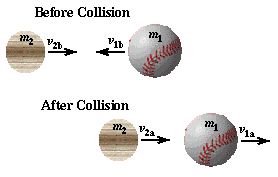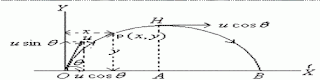## Posts

Showing posts from August, 2012

### Why a cricketer lowers his hands while catching a ball?We know how to calculate the kinetic energy of moving objects -- isn't that enough? No. It turns out that many situations involving collisions do not obey the simple conservation of Mechanical Energy. Why not? Because it takes energy to bend, break, mutilate and deform objects, energy which disappears from the kinetic and gravitational potential energy.
But a different quantity is conserved, even during collisions. The linear momentum of an object is defined as p = (mass) * (velocity) It is a vector quantity, and the total linear momentum of a bunch of objects will remain the same, before and after a collision.
Momentum is connected to force by impulse, which is simply impulse = (force) * (time) if the force has a constant magnitude during its action. If the force changes with time, then one must integrate to find the impulse: / impulse = | (force) dt / The Momentum-Impulse Theorem s…

### HEAVY OR LIGHT BAT?Momentum:

•Momentum is total quantity of motion possessed by body •  It is product of mass and velocity Collisions and the Conservation of Momentum          The impact between bat and ball is a collision between two objects, and in its simplest analysis the collision may be taken to occur in one-dimension. In reality most collisions between bat and ball (especially the ones I am able to make) are glancing collisions which require a two-dimensional analysis. It turns out, in fact, that a glancing blow is necessary to impart spin to the ball which allows it to travel farther. Maybe I'll write about this more interesting, but more difficult problem later, but for right now I'll keep things simple and look at the collision in one-dimension only. The ball, m1, and bat, m2, both have initial velocities before the collision (subscript "b"), with the ball's velocity being negative. After the collision (subscript "a") both bat and ball have positive velocities. …

### PROJECTILE MOTIONPROJECTILE •                       Projectile is the name given to a body thrown with some initial velocity, and then allowed to move in two dimensions under the action of gravity alone, without being propelled by any engine or fuel. The path followed by a projectile is called its trajectory. Examples of projectiles •       A cricket ball hit by a bat. •       A javelin or hammer thrown by an athlete.
•       A bullet fired from a rifle. •       A piece of stone thrown in any direction.
ASSUMPTIONS IN PROJECTILE MOTION
There is no frictional resistance of air. •       The effect due to rotation of earth and curvature of the earth is negligible. •       The acceleration due to gravity is constant in magnitude and direction at all points of the motion of projectile.
ANGULAR PROJECTION •       OX,is a horizontal line on ground and •        OY is a vertical line perpendicular to ground. •        Suppose a cricket ball be projected from the point O with velocity u, making an angle θ  with the horizontal…